Test: Gauss's Law

# Test: Gauss's Law

Test Description

## 10 Questions MCQ Test Electromagnetic Fields Theory (EMFT) | Test: Gauss's Law

Test: Gauss's Law for Electrical Engineering (EE) 2023 is part of Electromagnetic Fields Theory (EMFT) preparation. The Test: Gauss's Law questions and answers have been prepared according to the Electrical Engineering (EE) exam syllabus.The Test: Gauss's Law MCQs are made for Electrical Engineering (EE) 2023 Exam. Find important definitions, questions, notes, meanings, examples, exercises, MCQs and online tests for Test: Gauss's Law below.
Solutions of Test: Gauss's Law questions in English are available as part of our Electromagnetic Fields Theory (EMFT) for Electrical Engineering (EE) & Test: Gauss's Law solutions in Hindi for Electromagnetic Fields Theory (EMFT) course. Download more important topics, notes, lectures and mock test series for Electrical Engineering (EE) Exam by signing up for free. Attempt Test: Gauss's Law | 10 questions in 30 minutes | Mock test for Electrical Engineering (EE) preparation | Free important questions MCQ to study Electromagnetic Fields Theory (EMFT) for Electrical Engineering (EE) Exam | Download free PDF with solutions
 1 Crore+ students have signed up on EduRev. Have you?
Test: Gauss's Law - Question 1

### The Electric flux through the surfaceDetailed Solution for Test: Gauss's Law - Question 1

Gauss law:

• According to gauss law, for any enclosed surface the flux associated is 1/ϵo times the total charge enclosed within the surface.
• It is mathematically expressed as :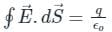• The flux associated with a surface is independent of the shape and size of the surface.
• It only depends on the number of charges enclosed within that surface.
• The surface should remain closed.
• Thus for any shape, if the number of charges enclosed within the surface is the same then the flux will also be the same.

Hence, the correct option is (4)

Test: Gauss's Law - Question 2

### Which of the following is correct about magnetic monopole?

Detailed Solution for Test: Gauss's Law - Question 2
• From Gauss law for magnetism, for a given surface the magnetic field lines entering the surface will be equal to the magnetic field lines leaving the surface. This is possible only when two poles are present.
• So, any magnetic field line entering will exit through the surface and any magnetic field exiting will enter through the surface. That means, there is no magnetic monopole where a magnetic field can start and end.
• ​Therefore, Gauss's law for magnetism concludes that magnetic monopoles do not exist.
• So, the pole of a magnet cannot be separated by breaking the magnet into two pieces.
Test: Gauss's Law - Question 3

### A point charge Q is located on the axis of a disk of radius R at a distance b from the plane of the disk as shown in below figure. Find the value of R, if 1/4th of the electric flux from the charge passes through the disk?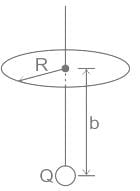Detailed Solution for Test: Gauss's Law - Question 3

Concept:
We know that the flux passing through a segment which makes angle θ at the center of the circle is: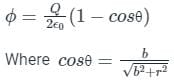Calculation:
Given if 1/4th of the flux passes through the disk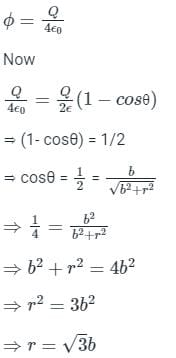Test: Gauss's Law - Question 4

The electric flux from a cube of side ‘a’ is ‘Φ’. What will be its value if the side of the cube is made ‘2a’ and the charge enclosed is made half?

Detailed Solution for Test: Gauss's Law - Question 4

CONCEPT:
Gauss's Law for electric field: It states that the total electric flux emerging out of a closed surface is directly proportional to the charge enclosed by this closed surface. It is expressed as:
ϕ = q/ϵ0
Where ϕ is the electric flux, q is the charge enclosed in the closed surface and ϵ0 is the electric constant.
Given that:
Consider a charge 'q' placed inside a cube of side 'a'.
The electric flux according to Gauss's law,
⇒ ϕ = q/ϵ0
If the charge enclosed is halved, then
⇒ q′ = q/2
Therefore, the new electric flux associated with this,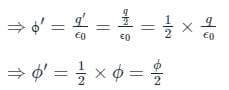Test: Gauss's Law - Question 5

The equation ∮E.dA = Q/εo represents __________.

Detailed Solution for Test: Gauss's Law - Question 5

Concept:

• Gauss' Law: This law gives the relation between the distribution of electric charge and the resulting electric field.
• According to this law, the total charge Q enclosed in a closed surface is proportional to the total flux ϕ  enclosed by the surface.

ϕ α Q

• The Gauss law formula is expressed by

ϕ = Q / ϵ0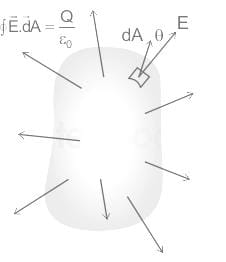Here,
Q = charge enclosed by the surface
ϵ0 = permittivity of free space
From the above explanation, we can see that according to Gauss law
ϕ = Q/ε0
Whereas, electric flux through small area dA is the product of the electric field and the area its passing through (dA)
∴ϕ = ∮E.dA = Q/ε0
Thus the given equation represents Gauss’s Law for electricity

Test: Gauss's Law - Question 6

According to gauss theorem, the electric flux on a closed surface depends on?

Detailed Solution for Test: Gauss's Law - Question 6

CONCEPT

• Electric Flux: It is defined as the number of electric field lines passing through the perpendicular unit area.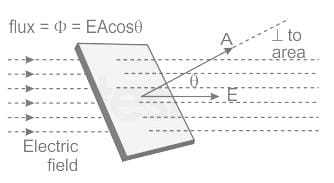• Electric Flux = (Φ) = EA [E = electric field, A = perpendicular area]
• Electric flux (Φ) = EA cos θ [where θ is the angle between area plane and electric field]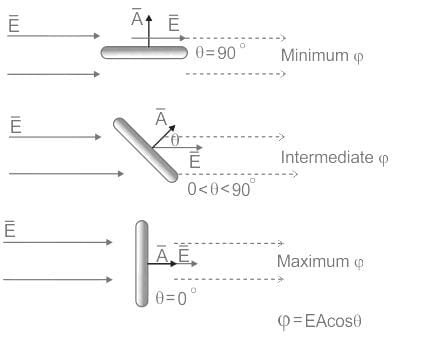• The flux is maximum when the angle is 0°
• Gauss Law: According to gauss’s law, total electric flux through a closed surface enclosing a charge is 1/ϵ0 times the magnitude of charge enclosed.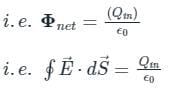Where, Φ = electric flux, Qin = charge enclosed the sphere, ϵ0 = permittivity of space (8.85 × 10-12 C2/Nm2), dS = surface area
According to gauss’s law,
The flux of the net electric field through a closed surface equals the net charge enclosed by the surface divided by ϵ0.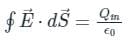Electric flux on a closed surface only depends on the enclosed charge.
∴ Option 2 is correct

Test: Gauss's Law - Question 7

The net electric flux through a spherical surface having 10 μC charge at the centre is

Detailed Solution for Test: Gauss's Law - Question 7

CONCEPT:

• Gauss's Law for electric field: It states that the total electric flux emerging out of a closed surface is directly proportional to the charge enclosed by this closed surface. It is expressed as:

ϕ = q/ϵ0
Where ϕ is the electric flux, q is the charge enclosed in the closed surface and ϵ0 is the permittivity of free space
The charge, q = 10 μC = 10 × 10-6 C
From Gauss's law, electric flux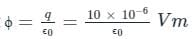Test: Gauss's Law - Question 8

If a unit positive charge is placed inside a sphere of radius r, then the electric flux through the sphere will be:

Detailed Solution for Test: Gauss's Law - Question 8

CONCEPT:
Gauss's law:

• According to Gauss law, the total electric flux linked with a closed surface called Gaussian surface is 1/ϵo the charge enclosed by the closed surface.

⇒ ϕ = Q/ϵo
Where ϕ = electric flux linked with a closed surface, Q = total charge enclosed in the surface, and ϵo = permittivity
Given Q = 1, and r = radius of the sphere
​By the Gauss law, if the total charge enclosed in a closed surface is Q, then the total electric flux associated with it will be given as,
⇒ ϕ = Q/ϵo     -----(1)
By equation 1 the total flux linked with the sphere is given as,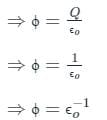Hence, option 3 is correct.

Test: Gauss's Law - Question 9

The total electric flux through a closed surface in which a certain amount of charge is placed depends on the:

Detailed Solution for Test: Gauss's Law - Question 9

CONCEPT:
Gauss's law:
According to Gauss law, the total electric flux linked with a closed surface called Gaussian surface is 1/ϵo the charge enclosed by the closed surface.
⇒ ϕ = Q/ϵo
Where ϕ = electric flux linked with a closed surface, Q = total charge enclosed in the surface, and ϵo = permittivity

Gauss's law:

• According to Gauss law, the total electric flux linked with a closed surface called Gaussian surface is 1/ϵo the charge enclosed by the closed surface.
• So if the total charge enclosed in a closed surface is Q, then the total electric flux associated with it will be given as,

⇒ ϕ = Qϵo   -----(1)

• By equation 1 it is clear that the total flux linked with the closed surface in which a certain amount of charge is placed does not depend on the shape and size of the surface. Hence, option 4 is correct.
Test: Gauss's Law - Question 10

The charge enclosed in the two cubes A and B is q. If ratio of the surface area of cubes A to cube B is 1 : 2, then the ratio of the flux associated with cube A to cube B will be:

Detailed Solution for Test: Gauss's Law - Question 10

Concept:
Gauss's law:

According to Gauss law, the total electric flux linked with a closed surface called Gaussian surface is 1/ϵo the charge enclosed by the closed surface.
⇒ ϕ = Q/ϵo
Where ϕ = electric flux linked with a closed surface, Q = total charge enclosed in the surface, and ϵo = permittivity

Calculation:
Given QA = QB = q, and AA : AB = 1 : 2
Where AA and AB = surface area of the cube A and the cube B respectively
By the Gauss law, if the total charge enclosed in a closed surface is Q, then the total electric flux associated with it will be given as,
⇒ ϕ = Q/ϵo     -----(1)
By equation 1 it is clear that the total flux linked with the closed surface in which a certain amount of charge is placed does not depend on the shape and size of the surface.
By equation 1 the total flux associated with cube A is given as,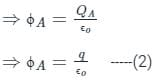By equation 1 the total flux associated with cube B is given as,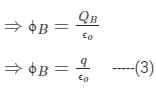By equation 2 and equation 3,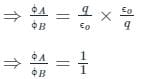Hence, option 3 is correct.

## Electromagnetic Fields Theory (EMFT)

11 videos|46 docs|62 tests
 Use Code STAYHOME200 and get INR 200 additional OFF Use Coupon Code
Information about Test: Gauss's Law Page
In this test you can find the Exam questions for Test: Gauss's Law solved & explained in the simplest way possible. Besides giving Questions and answers for Test: Gauss's Law, EduRev gives you an ample number of Online tests for practice

## Electromagnetic Fields Theory (EMFT)

11 videos|46 docs|62 tests